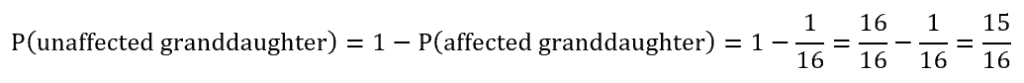# PCAT Quantitative Reasoning – Disease Inheritance Probability

A rare hereditary disease is passed on from mothers to daughters. A daughter has a 50% chance of inheriting this disease from an affected mother. If a woman with this disease has 6 grandchildren, each from a different child, what is the likelihood that none of them will be affected?##### Explanation

B is correct. Let’s draw a genetic pedigree to help us visualize this probability problem. We know that this woman is affected with the disease, so let’s shade in the symbol that represents her. She has six children, and each child has one of her six grandchildren. We know that there is a 50% or ½ probability that each child will be a girl, and if that is true, there is a ½ probability that that daughter will be affected. The same is true for the grandchildren: there is a ½ chance that each will be a girl, and then a ½ chance that each girl will be affected with the disease.The first step is to determine the probability that any given child is affected. Since “being a girl” and “being affected” can be considered two independent events occurring simultaneously, we should multiply the probability of each to determine the likelihood that each child is an affected daughter:Next, let’s use the same logic to calculate the probability of each grandchild being an affected daughter. For a grandchild to be affected, three conditions must be met: (1) she must be a girl; (2) she must be among the 50% who inherit the disease from an affected mother; and (3) she must have an affected mother. Recall that the probability of any of these grandchildren having an affected mother is ¼ from our previous calculation. Now let’s multiple the probabilities for each condition together:We’re almost done! What we have just calculated is the probability than any one of the grandchildren will be affected by this disease. What we want to determine is the probability that all of the grandchildren will not have the disease. The probability of an event not occurring is 1 minus the probability of that event:To find the probability that none of the grandchildren are affected, we must consider these independent events occurring simultaneously and multiply this probability by itself six times, once for each grandchild:#### Submit a Comment

This site uses Akismet to reduce spam. Learn how your comment data is processed.